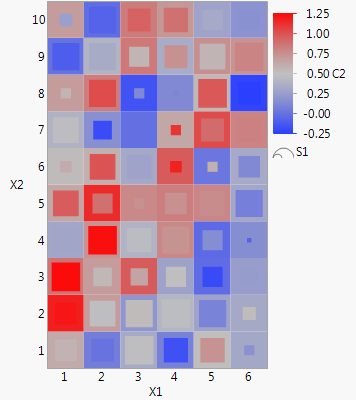Our World Statistics Day conversations have been a great reminder of how much statistics can inform our lives. Do you have an example of how statistics has made a difference in your life? Share your story with the Community!
Highlighted

## Glyph in Heatmap Display

Hi,

is there a way to render something more sophisticated in the heatmap display, such as a glyph.

The glyph would be positioned in the corresponding cell and would allow to see more values at the same time.

I'm thinking about an inner-outer rectangle glyph such as here (left most part)

Thanks Michael

Highlighted

## Re: Glyph in Heatmap Display

I was thinking it's not possible because Graph Builder can only have one color variable and doesn't overlay heat maps, but then I tried combining square markers with a heat map, and it creates the desired effect. I'm not sure if it works perceptually, but you can experiment with different size/marker combinations to see if can get your message across.

The main trick is to assign colors to the rows (with Rows > Color or Mark by Column) and tell the Points element in Graph Builder to ignore the variable in the color role (Properties > Points > Variables > untick C2) so only the heat map element uses it. Script below.`New Table( "double heat",  New Column( "X1", Nominal, Set Values( Mod( 0 :: 59, 6 ) + 1 ) ),  New Column( "X2", Nominal, Set Values( Floor( (0 :: 59) / 6 ) + 1 ) ),  New Column( "S1", Formula( Random Uniform() ) ),  New Column( "C1", Formula( Random Uniform() ) ),  New Column( "C2", Formula( :C1 + Random Normal() / 5 ) ));Current Data Table() << Run Formulas();// change the rows to use square markers (could be anything)For Each Row( Row State( Row() ) = Marker State( "Filled Square" ) );// Color each row marker based on column C1Current Data Table() << Color by Column( :C1, Color Theme( "Blue to Gray to Red" ) );Graph Builder(  Size( 257, 422 ), Show Control Panel( 0 ),  Variables( X( :X1 ), Y( :X2 ), Color( :C2 ), Size( :S1 ) ),  Elements(  Heatmap( X, Y, Legend( 13 ) ),  Points( X, Y, Color( 0 ), Legend( 12 ) )  ),  SendToReport( Dispatch( {}, "400", ScaleBox,  {Legend Model( 12, Properties( 0, {Marker Size( 6 )} ) )}  ) ))`
Article Labels

There are no labels assigned to this post.